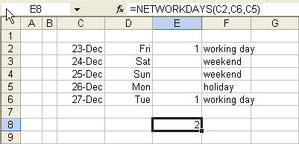# Excel Date functions or Date formulas

One of the pre-requisite to master the use of Date Functions or Date Formulas or in Excel is to know how Excel stores dates and times in the worksheets. This knowledge will go a long way to help you apply the more powerful date functions in Excel.

## Excel stores dates as numbers

Excel stores dates as numbers (specifically integers) or sequential

serial number in the worksheet. The date 1 Jan 2006 has a numerical value of 38718 while that for 1 Jan 1900 has the value of 1. By now, you should have figured out that the number presents the number of days the date is from 1 Jan 1900. 1 Jan 2006 is therefore 38,718 days from 1 Jan 1900. The interpretation is true only for dates on and after 1 Mar 1900. Click here for more information.

## Excel stores times as decimals

What about time? Excel stores time as decimals. 1 day is equal to 24 hours. Therefore, 12 hours is equal to 0.5 (12 hours divided by 24 hours) or 0.5 day. On the same basis, 3 hours is equivalent to 0.125, computed by taking 3 divided by 24. Therefore, if you are to enter the number 38718.125 into a cell and format the cell to show the date time format, it will show 1 Jan 2006, 3 am.

Now that we have a better understanding of dates and time in Excel, we can start off with our dates functions.

Date Function – Date(year, month, day)

What it does – Returns the sequential serial number that represents a particular date, i.e. show the sequential serial number (38718) of a particular date (1 Jan 2006)

After you have understood how Excel stores date and time, you might think this is a useless. It is actually not.

Consider a case when you exported the data records from a database with the year, month and day stored in different columns. You would not be able to format the date in Excel to show it in dd-mm-yy or any date format you want.

In this case, the date function would come in handy because you could convert it into an Excel recognizable date. Assuming that the Year is in Cell A1, Month in Cell B1 and Day in Cell C1. You can convert these value into a date by using the formula “=Date(A1,B1,C1)” (excluding the quote) in cell D1. After the conversion, you are able to perform other functions with the new value.

Date Functions � DAY(Serial_number), MONTH(Serial_number),YEAR(Serial_number)

What it does � Returns the day, month and year of a date (represented by a serial number)

Assuming you have a set of records and one of the fields is the date of birth. And you want to find out how many of these birthdays are in a particular month. How are you going to find out?

If you are to sort the dates, you would notice that the dates are presented from the earliest to the latest. You will never be able to sort them according to the month. This is because the dates are actually stored as serial numbers. (Click here to this section of the Date Functions for explanation). In order to sort the dates by their respective months, you need to use the MONTH Function. Assuming that the date of birth is in column A starting from 2nd row, then the formula is “=month(A2)” in row 2, “month(A3)”,…etc. Once you have the month of the birth date presented, you are able to group them with the SORT function.

Networkdays function – Networkdays(Start_date, End date,list of holidays)

You must activate the analysis Toolpak before you use this formula:

What it does – Calculates the number of working days between 2 dates

As the name implies, Networkdays function calculates the number of working days between 2 dates. The formula does not count weekends and holidays (as defined by the user). For example, to calculate the number of working days between 22 Dec (Fri) and 27 Dec (Wed), we can apply the networkdays formula in the following manner:C2 (23 Dec) refers to the start date, C6 (27 Dec) refers to the end date while C5 (26 Dec) refers to the holiday. The formula returns 2 working days because only Friday and Tuesday are working days. Monday is designated as a holiday in our formula. 24 Dec and 25 Dec are also not counted because they fall on weekend.

I will share with you more date functions in time to come. Do come back from time to time.

Have your say about what you just read! Leave me a comment in the box below.